## Example Questions

2 Next →

### Example Question #11 : Coordinate Geometry

The coordinates of the endpoints of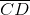, in the standard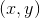coordinate plane, are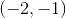and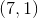. What is the-coordinate of the midpoint of?Explanation:

To answer this question, we need to find the midpoint of.

To find how far the midpoint of a line is from each end, we use the following equation: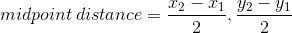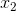and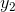are taken from the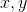value of the second point and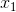and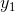are taken from thevalue of the first point. Therefore, for this data: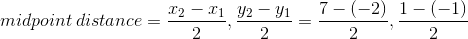We can then solve: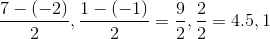Therefore, our midpoint is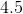units between each endpoint'svalue andunit between each endpoint'svalue. To find out the location of the midpoint, we subtract the midpoint distance from the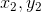point. (In this case it's the point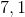.) Therefore: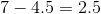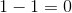So the midpoint is located at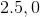The question asked us what the-coordinate of this point was. Therefore, our answer is.

### Example Question #12 : Coordinate Geometry

Following the line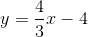, what is the distance from the the point whereto the point where?Explanation:

The first step is to find the y-coordinates for the two points we are using. To do this we plug our x-values into the equation. Where, we get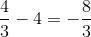, giving us the point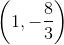. Where, we get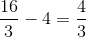, giving us the point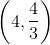.

We can now use the distance formula: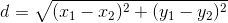.

Plugging in our points gives us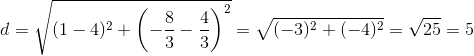### Example Question #13 : Coordinate Geometry

Which of the following is the slope-intercept form of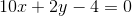?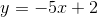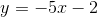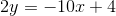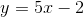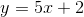Explanation:

To answer this question, we must put the equation into slope-intercept form, meaning we must solve for. Slope-intercept form follows the formatwhereis the slope andis the intercept.

Therefore, we must solve the equation so thatis by itself. First we addto both sides so that we can start to get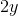by itself: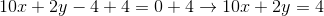Then, we must subtract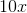from both sides: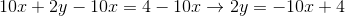We then must divide each side by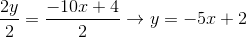Therefore, the slope-intercept form of the original equation is.

2 Next →